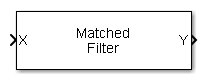# Matched Filter

Matched filter

## Library

Detection

`phaseddetectlib`

•## Description

The Matched Filter block implements matched filtering of an input signal. Matched filtering is an FIR filtering operation with the coefficients equal to the time reversed samples of the transmitted signal. The filter can improve SNR before detection.

## Parameters

Source of coefficients

Specify whether the matched filter coefficients come from Coefficients or from an input port.

 `Property` Matched filter coefficients are specified by Coefficients. `Input port` Matched filter coefficients are specified via the input port `Coeff`.
Coefficients

Specify the matched filter coefficients as a column vector. This parameter appears when you set Source of coefficients to `Property`.

Spectrum window

Specify the window used for spectrum weighting using one of

 `None` `Hamming` `Chebyshev` `Hann` `Kaiser` `Taylor`

Spectrum weighting is often used with linear FM waveforms to reduce sidelobe levels in the time domain. The block computes the window length internally to match the FFT length.

Spectrum window range

This parameter appears when you set the Spectrum window parameter to any value other than `None`. Specify the spectrum region, in hertz, on which the spectrum window is applied as a 1-by-2 vector in the form of `[StartFrequency,EndFrequency]`.

Note that both `StartFrequency` and `EndFrequency` are measured in baseband. That is, they are within `[-Fs/2,Fs/2]`, where `Fs` is the sample rate specified in any of the waveform library blocks. The parameter `StartFrequency` must be less than `EndFrequency`.

Sidelobe attenuation level

This parameter appears when you set Spectrum window to `Chebyshev` or `Taylor`. Specify the sidelobe attenuation level, in dB, of a Chebyshev or Taylor window as a positive scalar.

Kaiser shape parameter

This parameter appears when you set the Spectrum window parameter to `Kaiser`. Specify the parameter that affects the Kaiser window sidelobe attenuation as a nonnegative scalar. Please refer to the function `kaiser` for more details.

Number of constant level sidelobes

This parameter appears when you set the Spectrum window parameter to `Taylor`. Specify the number of nearly-constant-level sidelobes adjacent to the mainlobe in a Taylor window as a positive integer.

Enable SNR gain output

Select this check this box to obtain the matched filter SNR gain via the output port `G`. The output port appears only when this box is selected.

Simulate using

Block simulation method, specified as `Interpreted Execution` or ```Code Generation```. If you want your block to use the MATLAB® interpreter, choose `Interpreted Execution`. If you want your block to run as compiled code, choose `Code Generation`. Compiled code requires time to compile but usually runs faster.

Interpreted execution is useful when you are developing and tuning a model. The block runs the underlying System object™ in MATLAB. You can change and execute your model quickly. When you are satisfied with your results, you can then run the block using ```Code Generation```. Long simulations run faster than they would in interpreted execution. You can run repeated executions without recompiling. However, if you change any block parameters, then the block automatically recompiles before execution.

When setting this parameter, you must take into account the overall model simulation mode. The table shows how the Simulate using parameter interacts with the overall simulation mode.

When the Simulink® model is in `Accelerator` mode, the block mode specified using Simulate using overrides the simulation mode.

Acceleration Modes

 Block Simulation Simulation Behavior `Normal` `Accelerator` `Rapid Accelerator` `Interpreted Execution` The block executes using the MATLAB interpreter. The block executes using the MATLAB interpreter. Creates a standalone executable from the model. `Code Generation` The block is compiled. All blocks in the model are compiled.

## Ports

Note

The block input and output ports correspond to the input and output parameters described in the `step` method of the underlying System object. See link at the bottom of this page.

Port Supported Data Types
`X`

Input signal matrix.

The size of the first dimension of the input matrix can vary to simulate a changing signal length. A size change can occur, for example, in the case of a pulse waveform with variable pulse repetition frequency.

Double-precision floating point
`Coeff`

Matched filter coefficients.

Double-precision floating point
`Y`

Filter output.

Double-precision floating point
`G`

Matched-filter gain.

Double-precision floating point